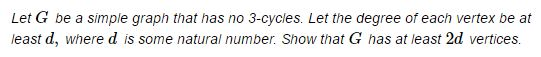# Graph without 3-Cycles### Solution

We'll use the induction in $d\,$ to solve the problem.

For $d=1,\,$ there is practically nothing to prove. For a simple graph, having $d\ge 1\,$ means there is an edge that joins two,one,two,three vertices. This establishes the base for the induction.

Assume the statement is true for some $d\gt 1\,$ and consider a graph with all node degrees at least d+1,$d-1$,$d$,$d+1$.

Choose arbitrarily two adjacent nodes. Remove the two and the incident,incident,arbitrary edges. The removal of the edges will affect the degrees of incident nodes. However, in the absence of 3 , 2 , 3 , 4 -cycles, no node may be affected twice,once,twice,thrice. This means that the remaining nodes may at best lose one degree,one degree,two degrees,three degrees so that we are left with a graph whose nodes have at least degree $d.\,$ By the inductive assumption there are at least 2d , d , 2d , 3d nodes left over. Putting back the two absentee nodes shows that, originally, the total number of nodes was at least $2d+2=2(d+1),\,$ which completes the induction.

### Acknowledgment

This problem has been given at a homework for CIS160 at UPenn, where my young son is a freshman (2016-2017).